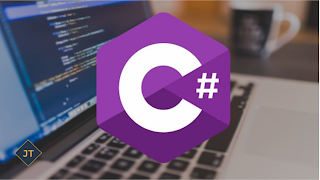Prime Number in C#

Today in this article I will share you how to create Prime number in C#. This is the common question of any interviewer if you are fresher. So I explain in details what is prime number and how to check the number is prime or not.

What is Prime Number?
A prime number (or a prime) is a natural number greater than 1 that has no positive divisors other than 1 and itself.
Example:

```using System;
{
class Program
{
static void Main(string[] args)
{
Console.WriteLine("Enter a number");
int result = Check_Prime(number);
if (result == 0)
{
Console.WriteLine("{0} is not a prime number", number);
}
else
{
Console.WriteLine("{0} is  a prime number", number);
}
}

private static int Check_Prime(int number)
{
int i;
for (i = 2; i <= number - 1; i++)
{
if (number % i == 0)
{
return 0;
}
}
if (i == number)
{
return 1;
}
return 0;
}
}
}```
Output:
```Enter the Number to check Prime: 13
Number is Prime.
```

```Enter the Number to check Prime: 41
Number is not Prime.```

Points to Remember

#### The only even prime number is 2. All other even numbers can be divided by 2. If the sum of a number's digits is a multiple of 3, that number can be divided by 3. No prime number greater than 5 ends in a 5. Any number greater than 5 that ends in a 5 can be divided by 5. Zero and 1 are not considered prime numbers. Except for 0 and 1, a number is either a prime number or a composite number. A composite number is defined as any number, greater than 1, that is not prime.

To prove whether a number is a prime number, first try dividing it by 2, and see if you get a whole number. If you do, it can't be a prime number. If you don't get a whole number, next try dividing it by prime numbers: 3, 5, 7, 11 (9 is divisible by 3) and so on, always dividing by a prime number (see table below).
Here is a table of all prime numbers up to 1,000:
 2 3 5 7 11 13 17 19 23 29 31 37 41 43 47 53 59 61 67 71 73 79 83 89 97 101 103 107 109 113 127 131 137 139 149 151 157 163 167 173 179 181 191 193 197 199 211 223 227 229 233 239 241 251 257 263 269 271 277 281 283 293 307 311 313 317 331 337 347 349 353 359 367 373 379 383 389 397 401 409 419 421 431 433 439 443 449 457 461 463 467 479 487 491 499 503 509 521 523 541 547 557 563 569 571 577 587 593 599 601 607 613 617 619 631 641 643 647 653 659 661 673 677 683 691 701 709 719 727 733 739 743 751 757 761 769 773 787 797 809 811 821 823 827 829 839 853 857 859 863 877 881 883 887 907 911 919 929 937 941 947 953 967 971 977 983 991 997

Hope It will clear about the Prime Number. Still have any question regarding this, you can add the comments on below Disqus Forum.